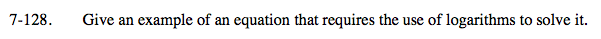Home > A2C > Chapter 7 > Lesson 7.2.3 > Problem7-128

7-128.

Give an example of an equation that requires the use of logarithms to solve it. Homework Help ✎Remember that equations with variables in the exponent that do not have common bases require logarithms to solve.